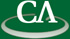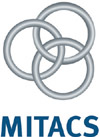## Normal Forms of Polynomial Matrices

### Howard Cheng, Department of Mathematics and Computer Science, University of Lethbridge

Wednesday June 4th, 2008 at 3:30pm in K9509.

```Abstract:

Normal forms for polynomial matrices are used in applications such as
control theory.  The Hermite form, row-reduced form, weak Popov form
and Popov form are introduced.  It is shown how these normal forms can
be computed by straightforward elimination-based algorithms.  In many
cases, elimination-based approaches are insufficient because of
intermediate expression swell on the coefficients.  Fraction-free and
modular algorithms for these problems are briefly outlined.

```